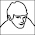1 Feb 2012

Transformation of Several Variables in a Dataframe

This is how I transform several columns of a dataframe, i.e., with count-data into binary coded data (this would apply also for any other conversion..).

```count1 <- count2 <- count3 <- count4 <- sample(c(rep(0, 10), 1:10))
some <- LETTERS[1:20]
thing <- letters[1:20]
mydf <- data.frame(count1, count2, count3, count4, some, thing)

ids <- grep("count", names(mydf))
myfun <- function(x) {ifelse(x > 0, 1, 0)}
mydf[, ids] <- lapply(mydf[, ids], myfun)
```

p.s.: Let me know if you know of a slicker way.

1.There is a typo in line 4 ('data.frame').

Another possibility for a presence-absence transformation would be vegan::decostand() :

mydf[, ids] <- decostand(mydf[, ids], method = "pa")

1.Edi, many thanks for the pointer!

2.set.seed(1)
x <- sample(0:100, 1E7, replace=TRUE, prob=c(0.5, rep(0.005, 100)))
system.time(ifelse(x > 0, 1, 0))
user system elapsed
7.092 2.488 9.634
system.time(as.numeric(x > 0))
user system elapsed
0.344 0.280 0.629

For real biological datasets this is probably trivial (10 million records is perhaps far-fetched!).

1.Thanks John!